## Measure

The terms measure,'' measurable,'' etc., have very precise technical definitions (usually involving Sigma Algebras) which makes them a little difficult to understand. However, the technical nature of the definitions is extremely important, since it gives a firm footing to concepts which are the basis for much of Analysis (including some of the slippery underpinnings of Calculus).

For example, every definition of an Integral is based on a particular measure: the Riemann Integral is based on Jordan Measure, and the Lebesgue Integral is based on Lebesgue Measure. The study of measures and their application to Integration is known as Measure Theory.

A measure is formally defined as a Nonnegative Map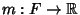(the reals) such that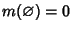and, if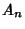is a Countable Sequence in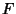and theare pairwise Disjoint, then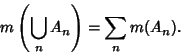If, in addition,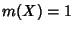, thenis said to be a Probability Measure.

A measuremay also be defined on Sets other than those in the Sigma Algebra. By adding toall sets to whichassigns measure zero, we again obtain a Sigma Algebra and call this the completion'' ofwith respect to. Thus, the completion of a Sigma Algebra is the smallest Sigma Algebra containingand all sets of measure zero.

See also Almost Everywhere, Borel Measure, Ergodic Measure, Euler Measure, Gauss Measure, Haar Measure, Hausdorff Measure, Helson-Szegö Measure, Integral, Jordan Measure, Lebesgue Measure, Liouville Measure, Mahler's Measure, Measurable Space, Measure Algebra, Measure Space, Minkowski Measure, Natural Measure, Probability Measure, Wiener Measure